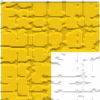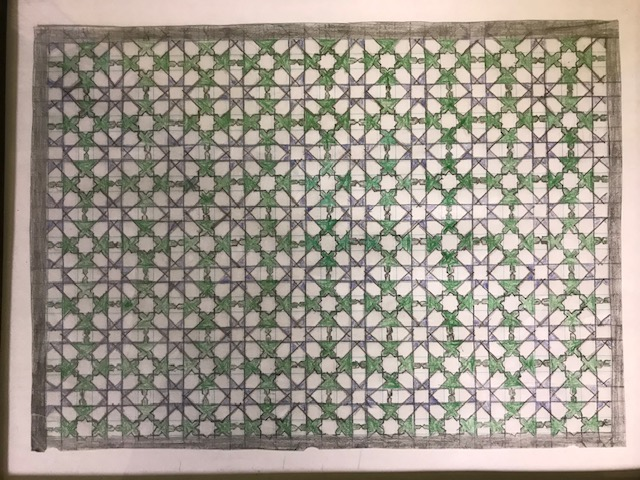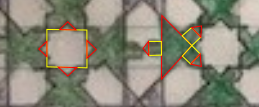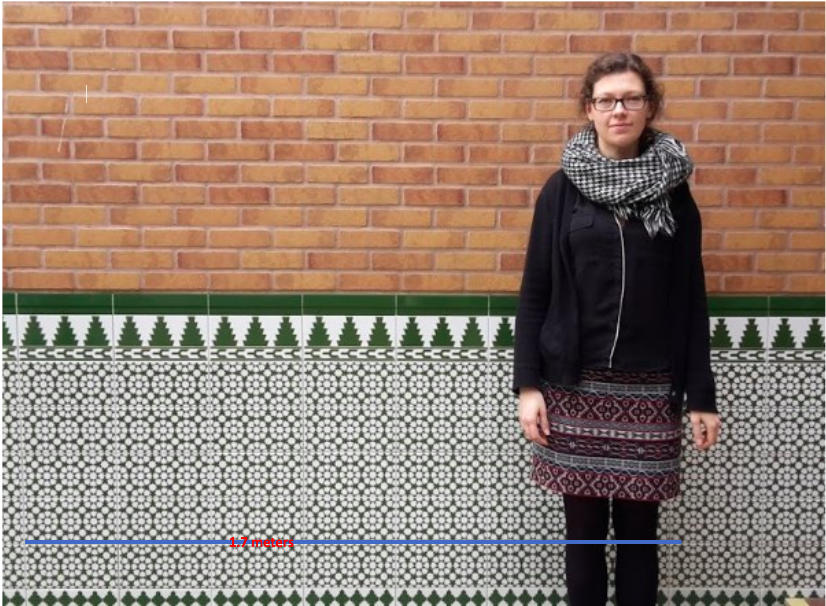#### You may also like### Triominoes

A triomino is a flat L shape made from 3 square tiles. A chess board is marked into squares the same size as the tiles and just one square, anywhere on the board, is coloured red. Can you cover the board with trionimoes so that only the square is exposed?### LOGO Challenge 5 - Patch

Using LOGO, can you construct elegant procedures that will draw this family of 'floor coverings'?### LOGO Challenge - Triangles-squares-stars

Can you recreate these designs? What are the basic units? What movement is required between each unit? Some elegant use of procedures will help - variables not essential.

# Gibraltar Geometry

##### Age 11 to 14Challenge Level

How many lines of symmetry are there?
What is the ratio of green to white?
What area do the patterns cover?
Is there a specific name for a pattern that repeats in this way?

Can you answer any of them?

Darcy from St Stephens School in Australia found 350 8-pointed stars in the mosaic.

Kushan from Southbury Elementary School in the USA described the shapes in a different way, and recreated the pattern.
I think it's made up of squares and triangles because the octagonal stars have four triangles at the edge and the "froggy" shapes have a big triangle with three squares poking out of the sides with triangles at the edges. Please see my drawing to locate the shapes.Here are the squares and triangles Kushan describes:Abigail and Bella from St Stephens Carramar in Australia also sent in their work:
We are trying to figure out the size of the tiles:
Each tile is 24cm long. We did this by drawing a line from her head to her toes (where we predicted they would be).
We knew she was 1.7 meters tall so, so we rotated the line we had drawn and counted how many tiles were fitted along the line: 7
So we divided 1.7 by 7 and we got 24 cm.JEloise, also from St Stephens Carramar, posed and answered a different question using Becky:
How much space does Becky take up on the tiled wall?

Step 1: Find someone/something that measures 1.7 m
Step 2: Measure from their elbows to their ankles
*Becky takes up $2 \frac12$ average sized bricks*
Step 3: Measure the length of $2 \frac12$ average sized bricks (that equals her width)
Step 4: Length $\times$ width = her estimated area

Step 5: Find out the length of elbows to just above the knees
Step 6: Height from above the knees to the elbows x overall width *we use
that number later*
Step 7: Figure out the height from above the knees to the ankles
Step 8: Add step 6 & 7 number together = estimated area Becky takes up

*the next few steps is how to make it more accurate. If you're not interested in it, stop here and you'll have the rough answer of how much space she takes up*

Step 9: Figure out the measurement of both legs combined *including the gap*
Step 10: figure out measurements of both size gap *top and bottom*
Step 11: Subtract gap size from the leg measurements. = How much space Becky takes up on the tiled wall.

This is how we got our final answer.

*we had a friend that was exactly 1.7m so it made it easy to predict Becky's size, this formula may not work for everyone*